## Calculus

 Question 1

If f(1) = 2, f(2) = 4 and f(4) = 16, what is the value of f(3) using Lagrange’s interpolation formula?

 A 8 B 8(1/3) C 8(2/3) D 9
Question 1 Explanation:
Note: Out of syllabus.
 Question 2

Consider the following iterative root finding methods and convergence properties:

```Iterative root finding          Convergence properties methods
(Q) False Position                (I) Order of convergence = 1.62
(R) Newton Raphson               (II) Order of convergence = 2
(S) Secant                      (III) Order of convergence = 1
with guarantee of convergence
(T) Successive Approximation     (IV) Order of convergence = 1
with no guarantee of convergence ```
 A Q-II, R-IV, S-II, T-I B Q-III, R-II, S-I, T-IV C Q-II, R-I, S-IV, T-III D Q-I, R-IV, S-II, T-III
Question 2 Explanation:
Note: Out of syllabus.
 Question 3

Consider the functions

I. e-x
II. x2-sin x
III. √(x3+1)

Which of the above functions is/are increasing everywhere in [0,1]?

 A II and III only B III only C II only D I and III only
Question 3 Explanation:
A function f(x) is said to be increasing if f'(x)>0 at each point in an interval.
I. e-x
II. f'(x) = -e-x
f'(x)<0 on the interval [0,1] so this is not an increasing function.
II. x2-sinx
f'(x) = 2x - cosx
at x=0, f'(0) = 2(0) - 1 = -1 < 0
f(x) = x2 - sinx is decreasing over some interval, increasing over some interval as cosx is periodic.
III. √(x3+1) = (x3+1)1/2
f'(x) = 1/2(3x2/√(x3+1))>0
f(x) is increasing over [0,1].
 Question 4
The value of the following limit is _____________.A 1/2
Question 4 Explanation:When 0 is substituted, we get 0/0
Apply L- Hospital rule-1/2
 Question 5

The formula used to compute an approximation for the second derivative of a function f at a point X0 is

 A f(x0+h) + f(x0-h)/2 B f(x0+h) - f(x0-h)/2h C f(x0+h) + 2f(x0) + f(x0-h)/h2 D f(x0+h) - 2f(x0) + f(x0-h)/h2
Question 5 Explanation:
The formula which is used to compute the second derivation of a function f at point X is
f(x0+h) - 2f(x0) + f(x0-h)/h2
 Question 6

What is the maximum value of the function f(x) = 2x2 - 2x + 6 in the interval [0,2]?

 A 6 B 10 C 12 D 5.5
Question 6 Explanation:
For f(x) to be maximum
f'(x) = 4x - 2 = 0
⇒ x = 1/2
So at x = 1/2, f(x) is an extremum (either maximum or minimum).
f(2) = 2(2)2 - 2(2) + 6 = 10
f(1/2) = 2 × (1/2)2 - 2 × 1/2 + 6 = 5.5
f(0) = 6
So, the maximum value is at x=2 which is 10 as there are no other extremum for the given function.
 Question 7

Consider the function y = |x| in the interval [-1,1]. In this interval, the function is

 A continuous and differentiable B continuous but not differentiable C differentiable but not continuous D neither continuous nor differentiable
Question 7 Explanation:
The given function y = |x| be continuous but not differential at x= 0.
→ The left side values of x=0 be negative and right side values are positive.
→ If the function is said to be differentiable then left side and right side values are to be same.
 Question 8

(a) Find the points of local maxima and minima, if any, of the following function defined in 0 ≤ x ≤ 6.

`  x3 - 6x + 9x - 15 `

(b) IntegrateA Theory Explanation.
 Question 9Which of the following statements is true?

 A S > T B S = T C S < T and 2S > T D 2S ≤ T
Question 9 Explanation:
S is continuously increasing function but T represent constant value so S>T.
 Question 10

The solution of differential equation y'' + 3y' + 2y = 0 is of the form

 A C1ex + C2e2x B C1e-x + C2e3x C C1e-x + C2e-2x D C1e-2x + C22-x
Question 10 Explanation:
Note: Out of syllabus.
 Question 11

(a) Determine the number of divisors of 600.
(b) Compute without using power series expansionA Theory Explanation.
 Question 12

Backward Euler method for solving the differential equation dy/dx = f(x,y) is specified by, (choose one of the following).

 A yn+1 = yn + hf(xn, yn) B yn+1 = yn + hf(xn+1, yn+1) C yn+1 = yn-1 + 2hf(xn, yn) D yn+1 = (1 + h) f(xn+1, yn+1)
Question 12 Explanation:
dy/dx = f(x,y)
With initial value y(x0) = y0. Here the function f and the initial data x0 and y0 are known. The function y depends on the real variable x and is unknown. A numerical method produces a sequence y0, y1, y2, ....... such that yn approximates y(x0 + nh) where h is called the step size.
→ The backward Euler method is helpful to compute the approximations i.e.,
yn+1 = yn + hf(x n+1, yn+1)
 Question 13

The differential equation
d2y/dx2 + dy/dx + siny = 0 is:

 A linear B non-linear C homogeneous D of degree two
Question 13 Explanation:
Note: Out of syllabus.
d2y/dx2 + dy/dx + siny = 0
In this DE, degree is 1 then this represent linear equation.
 Question 14

Fourier series of the periodic function (period 2π) defined by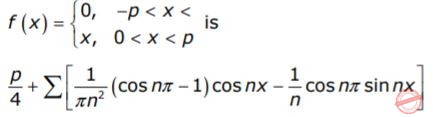But putting x = π, we get the sum of the series.A π2/4 B π2/6 C π2/8 D π2/12
Question 14 Explanation:
Note: Out of syllabus.
 Question 15

Which of the following improper integrals is (are) convergent?

 AB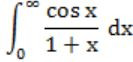CD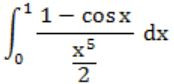Question 16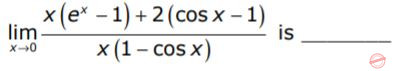A 1
Question 16 Explanation:
Since the given expression is in 0/0 form, so we can apply L-Hospital rule.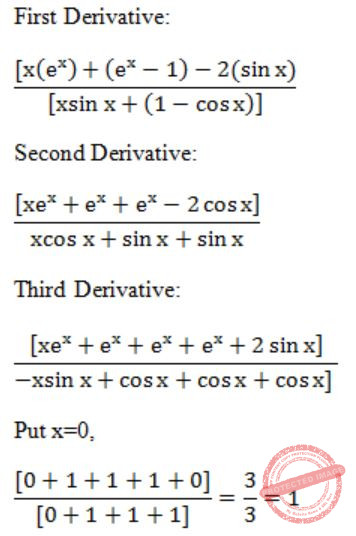Question 17

The radius of convergence of the power seriesA Out of syllabus.
 Question 18

The value of the double integral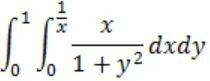is

 A 1/3
Question 18 Explanation:Question 19

The differential equation yn + y = 0 is subjected to the boundary conditions.

`    y (0) = 0        y(λ) = 0         `

In order that the equation has non-trivial solution(s), the general value of λ is __________

 A Out of syllabus.
 Question 20

If the trapezoidal method is used to evaluate the integral obtained 01x2dx ,then the value obtained

 A is always > (1/3) B is always < (1/3) C is always = (1/3) D may be greater or lesser than (1/3)
Question 20 Explanation:
Note: Out of syllabus.
 Question 21

What is the value ofA -1 B 1 C 0 D π
Question 21 Explanation:In the limits are be -π to π, one is odd and another is even product of even and odd is odd function and integrating function from the same negative value to positive value gives 0.
 Question 22
Consider the following expression.The value of the above expression (rounded to 2 decimal places) is _______
 A 0.25
Question 22 Explanation:Question 23

If f(x) is defined as follows, what is the minimum value of f(x) for x∊(0,2] ?A 2 B 2(1/12) C 2(1/6) D 2(1/2)
Question 23 Explanation:
If x = 3/2
f(x) = 25/8x = 25/8(3/2) = 25/12 = 2(1/12)
 Question 24

The following definite integral evaluates to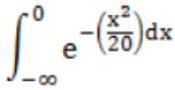A 1/2 B π √10 C √10 D π E None of the above
Question 24 Explanation:Question 25

Consider the following two statements about the function f(x)=|x|

```P. f(x) is continuous for all real values of x
Q. f(x) is differentiable for all real values of x ```

Which of the following is TRUE?

 A P is true and Q is false. B P is false and Q is true. C Both P and Q are true. D Both P and Q are false.
Question 25 Explanation:
f(x) = |x|
→ f(x) is continuous for all real values of xFor every value of x, there is corresponding value of f(x).
For x is positive, f(x) is also positive
x is negative, f(x) is positive.
So, f(x) is continuous for all real values of x.
→ f(x) is not differentiable for all real values of x. For x<0, derivative is negative
x>0, derivative is positive.
Here, left derivative and right derivatives are not equal.
 Question 26equals
 A 1 B -1 C ∞ D -∞
Question 26 Explanation:Question 27

A point on a curve is said to be an extremum if it is a local minimum or a local maximum. The number of distinct extrema for the curve 3x4 - 16x3 + 24x2 + 37 is

 A 0 B 1 C 2 D 3
Question 27 Explanation:
f(x) = 3x4 - 16x3 + 24x2 + 37
f’(x) = 12x3 + 48x2 + 48x = 0
12x(x2 - 4x + 4) = 0
x=0; (x-2)2 = 0
x=2
f’’(x) = 36x2 - 96x + 48
f ”(0) = 48
f ”(2) = 36(4) - 96(2) + 48
= 144 - 192 + 48
= 0
At x=2, we can’t apply the second derivative test.
f’(1) = 12; f’(3) = 36, on either side of 2 there is no sign change then this is neither minimum or maximum.
Finally, we have only one Extremum i.e., x=0.
 Question 28

Let f(x) = x -(1/3) and A denote the area of the region bounded bu f(x) and the X-axis, when x varies from -1 to 1. Which of the following statements is/are TRUE?

I) f is continuous in [-1,1]
II) f is not bounded in [-1,1]
III) A is nonzero and finite
 A II only B III only C II and III only D I, II and III
Question 28 Explanation:
Since f(0)→∞
∴ f is not bounced in [-1, 1] and hence f is not continuous in [-1, 1].∴ Statement II & III are true.
 Question 29

Let f(x) be a polynomial and g(x) = f'(x) be its derivative. If the degree of (f(x) + f(-x)) is 10, then the degree of (g(x) - g(-x)) is __________.

 A 9 B 10 C 11 D 12
Question 29 Explanation:
If the degree of a polynomial is ‘n’ then the derivative of that function have (n – 1) degree.
It is given that f(x) + f(-x) degree is 10.
It means f(x) is a polynomial of degree 10.
Then obviously the degree of g(x) which is f’(x) will be 9.
 Question 30A 4 B 3 C 2 D 1
Question 30 Explanation:Question 31

If f(x) = Rsin(πx/2) + S, f'(1/2) = √2 and, then the constants R and S are, respectively.

 A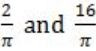B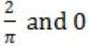C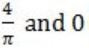DQuestion 31 Explanation: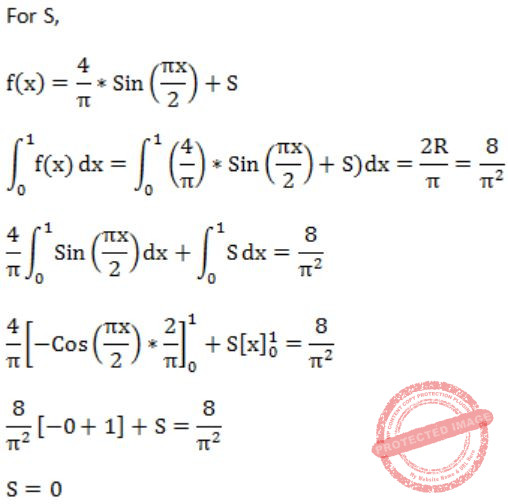Question 32
The value ofA is 0 B is -1 C is 1 D does not exist
Question 32 Explanation:If "x=1" is substituted we get 0/0 form, so apply L-Hospital ruleSubstitute x=1
⇒ (7(1)6-10(1)4)/(3(1)2-6(1)) = (7-10)/(3-6) = (-3)/(-3) = 1Question 33A 1 B Limit does not exist C 53/12 D 108/7
Question 33 Explanation:There are 33 questions to complete.

Register Now